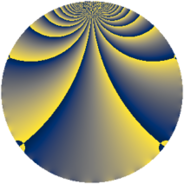# Properties

 Label 1520.2.tLevel $1520$ Weight $2$ Character orbit 1520.t Rep. character $\chi_{1520}(1027,\cdot)$ Character field $\Q(\zeta_{4})$ Dimension $432$ Sturm bound $480$

# Related objects

## Defining parameters

 Level: $$N$$ $$=$$ $$1520 = 2^{4} \cdot 5 \cdot 19$$ Weight: $$k$$ $$=$$ $$2$$ Character orbit: $$[\chi]$$ $$=$$ 1520.t (of order $$4$$ and degree $$2$$) Character conductor: $$\operatorname{cond}(\chi)$$ $$=$$ $$80$$ Character field: $$\Q(i)$$ Sturm bound: $$480$$

## Dimensions

The following table gives the dimensions of various subspaces of $$M_{2}(1520, [\chi])$$.

Total New Old
Modular forms 488 432 56
Cusp forms 472 432 40
Eisenstein series 16 0 16

## Trace form

 $$432q - 432q^{9} + O(q^{10})$$ $$432q - 432q^{9} - 16q^{12} - 16q^{22} + 16q^{26} + 32q^{28} - 24q^{30} + 40q^{32} + 16q^{34} - 40q^{40} - 80q^{42} + 64q^{43} - 64q^{44} - 84q^{48} + 60q^{52} + 16q^{54} - 48q^{56} - 32q^{58} + 32q^{59} + 92q^{60} - 24q^{62} + 16q^{66} - 96q^{68} + 24q^{70} - 64q^{71} + 100q^{72} - 16q^{73} - 56q^{74} - 32q^{75} + 128q^{78} - 16q^{80} + 432q^{81} - 24q^{82} + 16q^{86} + 108q^{88} + 136q^{90} - 32q^{91} - 40q^{92} + 16q^{96} - 88q^{98} + 32q^{99} + O(q^{100})$$

## Decomposition of $$S_{2}^{\mathrm{new}}(1520, [\chi])$$ into newform subspaces

The newforms in this space have not yet been added to the LMFDB.

## Decomposition of $$S_{2}^{\mathrm{old}}(1520, [\chi])$$ into lower level spaces

$$S_{2}^{\mathrm{old}}(1520, [\chi]) \cong$$ $$S_{2}^{\mathrm{new}}(80, [\chi])$$$$^{\oplus 2}$$